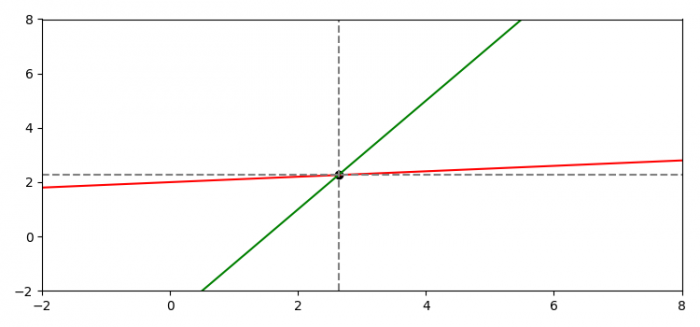# How do I find the intersection of two line segments in Matplotlib?

To find the intersection of two lines segments in Matplotlib and pass the horizontal and vertical lines through that point, we can take the following steps −

• Set the figure size and adjust the padding between and around the subplots.
• Create two lines using slopes (m1, m2) and intercepts (c1 and c2). Initialize the slopes and intercept values.
• Create x data points using numpy.
• Plot x, m1, m2, c2 and c1 data points using plot() method.
• Using intercepts and slope values, find the point of intersection.
• Plot the horizontal and vertical lines with dotted linestyle.
• Plot xi and yi points on the plot.
• To display the figure, use show() method.

## Example

import matplotlib.pyplot as plt
import numpy as np

plt.rcParams["figure.figsize"] = [7.50, 3.50]
plt.rcParams["figure.autolayout"] = True

m1, c1 = 0.1, 2.0
m2, c2 = 2.0, -3.0

x = np.linspace(-10, 10, 500)

plt.plot(x, x * m1 + c1, 'red')
plt.plot(x, x * m2 + c2, 'green')

plt.xlim(-2, 8)
plt.ylim(-2, 8)

xi = (c1 - c2) / (m2 - m1)
yi = m1 * xi + c1

plt.axvline(x=xi, color='gray', linestyle='--')
plt.axhline(y=yi, color='gray', linestyle='--')

plt.scatter(xi, yi, color='black')

plt.show()

## OutputUpdated on: 18-Jun-2021

5K+ Views